1、径向基函数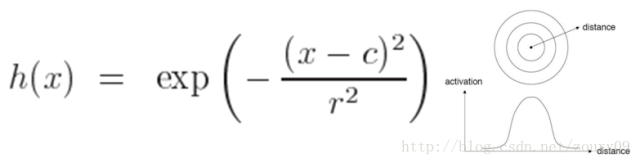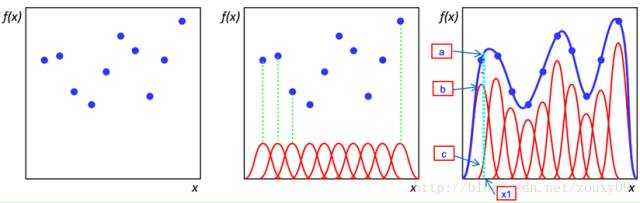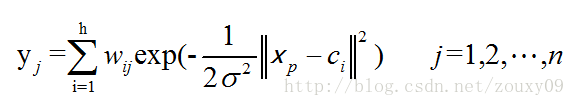RBF网络的结构与多层前向网络类似，它是一种三层前向网络。输入层由信号源结点组成；第二层为隐含层，隐单元数视所描述问题的需要而定，隐单元的变换函数是RBF径向基函数，它是对中心点径向对称且衰减的非负非线性函数；第三层为输出层，它对输入模式的作用作出响应。从输人空间到隐含层空间的变换是非线性的，而从隐含层空间到输出层空间变换是线性的。RBF网络的基本思想是：用RBF作为隐单元的“基”构成隐含层空间，这样就可将输入矢量直接（即不需要通过权连接）映射到隐空间。根据Cover定理，低维空间不可分的数据到了高维空间会更有可能变得可分。换句话来说，RBF网络的隐层的功能就是将低维空间的输入通过非线性函数映射到一个高维空间。然后再在这个高维空间进行曲线的拟合。它等价于在一个隐含的高维空间寻找一个能最佳拟合训练数据的表面。这点与普通的多层感知机MLP是不同的。

RBF的设计主要包括两个方面，一个是结构设计，也就是说隐藏层含有几个节点合适。另一个就是参数设计，也就是对网络各参数进行求解。由上面的输入到输出的网络映射函数公式可以看到，网络的参数主要包括三种：径向基函数的中心、方差和隐含层到输出层的权值。到目前为止，出现了很多求解这三种参数的方法，主要可以分为以下两大类：

1、方法一：

（1）在训练样本集中随机选择h个样本作为h个径向基函数的中心。更好的方法是通过聚类，例如K-means聚类得到h个聚类中心，将这些聚类中心当成径向基函数的h个中心。

（2）RBF神经网络的基函数为高斯函数时，方差可由下式求解：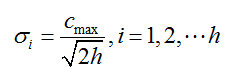（3）隐含层至输出层之间神经元的连接权值可以用最小均方误差LMS直接计算得到，计算公式如下：（计算伪逆）（d是我们期待的输出值）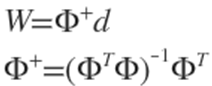2、方法二：

（1）随机初始化径向基函数的中心、方差和隐含层到输出层的权值。当然了，也可以选用方法一中的（1）来初始化径向基函数的中心。

（2）通过梯度下降来对网络中的三种参数都进行监督训练优化。代价函数是网络输出和期望输出的均方误差：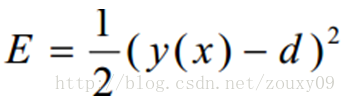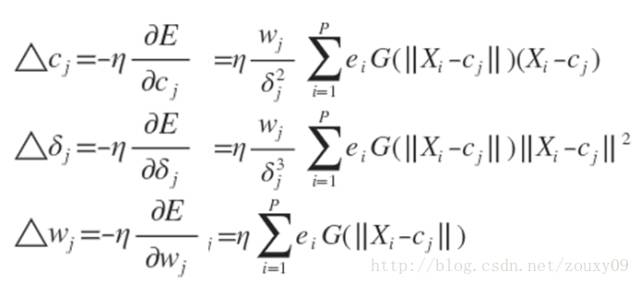1、第一种方法

Matlab提供的一个函数是newrb()。它有个技能就是可以自动增加网络的隐层神经元数目直到均方差满足我们要求的精度或者神经元数数目达到最大（也就是我们提供的样本数目，当神经元数目和我们的样本数目一致时，rbf网络此时的均方误差为0）为止。它使用方法也能简单：

rbf = newrb(train_x, train_y);

output = rbf(test_x);

2、第二种方法

learnRBF.m

[cpp] view plain copy

1. %// This is a RBF network trained by BP algorithm

2. %// Author : zouxy

3. %// Date   : 2013-10-28

4. %// HomePage : http://blog.csdn.net/zouxy09

5. %// Email  : zouxy09@qq.com

6. close all; clear; clc;

7. %%% ************************************************

8. %%% ************ step 0: load data ****************

9. display('step 0: load data...');

10. % train_x = [1 2 3 4 5 6 7 8]; % each sample arranged as a column of train_x

11. % train_y = 2 * train_x;

12. train_x = rand(5, 10);

13. train_y = 2 * train_x;

14. test_x = train_x;

15. test_y = train_y;

16. %% from matlab

17. % rbf = newrb(train_x, train_y);

18. % output = rbf(test_x);

19. %%% ************************************************

20. %%% ******** step 1: initialize parameters ********

21. display('step 1: initialize parameters...');

22. numSamples = size(train_x, 2);

23. rbf.inputSize = size(train_x, 1);

24. rbf.hiddenSize = numSamples;        % num of Radial Basis function

25. rbf.outputSize = size(train_y, 1);

26. rbf.alpha = 0.1;  % learning rate (should not be large!)

27. %% centre of RBF

28. for i = 1 : rbf.hiddenSize

29. % randomly pick up some samples to initialize centres of RBF

30. index = randi([1, numSamples]);

31. rbf.center(:, i) =  train_x(:, index);

32. end

33. %% delta of RBF

34. rbf.delta = rand(1, rbf.hiddenSize);

35. %% weight of RBF

36. r = 1.0; % random number between [-r, r]

37. rbf.weight = rand(rbf.outputSize, rbf.hiddenSize) * 2 * r - r;

38. %%% ************************************************

39. %%% ************ step 2: start training ************

40. display('step 2: start training...');

41. maxIter = 400;

42. preCost = 0;

43. for i = 1 : maxIter

44. fprintf(1, 'Iteration %d ,', i);

45. rbf = trainRBF(rbf, train_x, train_y);

46. fprintf(1, 'the cost is %d \n', rbf.cost);

47. curCost = rbf.cost;

48. if abs(curCost - preCost) < 1e-8

49. disp('Reached iteration termination condition and Termination now!');

50. break;

51. end

52. preCost = curCost;

53. end

54. %%% ************************************************

55. %%% ************ step 3: start testing ************

56. display('step 3: start testing...');

57. Green = zeros(rbf.hiddenSize, 1);

58. for i = 1 : size(test_x, 2)

59. for j = 1 : rbf.hiddenSize

60. Green(j, 1) = green(test_x(:, i), rbf.center(:, j), rbf.delta(j));

61. end

62. output(:, i) = rbf.weight * Green;

63. end

64. disp(test_y);

65. disp(output);

trainRBF.m

[cpp] view plain copy

1. function [rbf] = trainRBF(rbf, train_x, train_y)

2. %%% step 1: calculate gradient

3. numSamples = size(train_x, 2);

4. Green = zeros(rbf.hiddenSize, 1);

5. output = zeros(rbf.outputSize, 1);

6. delta_weight = zeros(rbf.outputSize, rbf.hiddenSize);

7. delta_center = zeros(rbf.inputSize, rbf.hiddenSize);

8. delta_delta =  zeros(1, rbf.hiddenSize);

9. rbf.cost = 0;

10. for i = 1 : numSamples

11. %% Feed forward

12. for j = 1 : rbf.hiddenSize

13. Green(j, 1) = green(train_x(:, i), rbf.center(:, j), rbf.delta(j));

14. end

15. output = rbf.weight * Green;

16. %% Back propagation

17. delta3 = -(train_y(:, i) - output);

18. rbf.cost = rbf.cost + sum(delta3.^2);

19. delta_weight = delta_weight + delta3 * Green';

20. delta2 = rbf.weight' * delta3 .* Green;

21. for j = 1 : rbf.hiddenSize

22. delta_center(:, j) = delta_center(:, j) + delta2(j) .* (train_x(:, i) - rbf.center(:, j)) ./ rbf.delta(j)^2;

23. delta_delta(j) = delta_delta(j)+ delta2(j) * sum((train_x(:, i) - rbf.center(:, j)).^2) ./ rbf.delta(j)^3;

24. end

25. end

26. %%% step 2: update parameters

27. rbf.cost = 0.5 * rbf.cost ./ numSamples;

28. rbf.weight = rbf.weight - rbf.alpha .* delta_weight ./ numSamples;

29. rbf.center = rbf.center - rbf.alpha .* delta_center ./ numSamples;

30. rbf.delta = rbf.delta - rbf.alpha .* delta_delta ./ numSamples;

31. end

green.m

[plain] view plain copy

1. function greenValue = green(x, c, delta)

2. greenValue = exp(-1.0 * sum((x - c).^2) / (2 * delta^2));

3. end

train_x = [1 2 3 4 5 6 7 8];

train_y = 2 * train_x;

2    4     6     8   10    12    14   16

2.0042   4.0239    5.9250    8.0214  10.0692   11.9351   14.0179  15.9958

Matlab的newrb的输出是：

2.0000   4.0000    6.0000    8.0000  10.0000   12.0000   14.0000  16.0000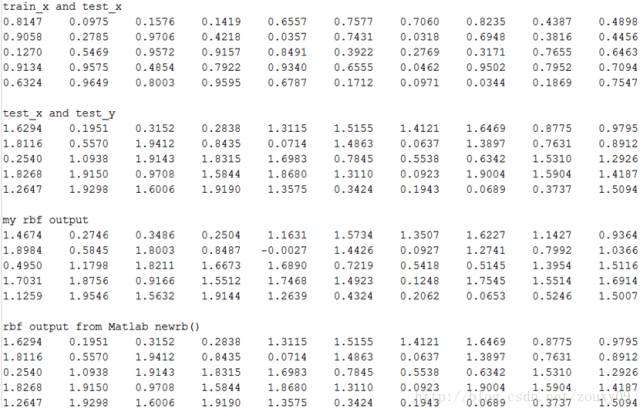## 径向基网络（RBF network）的更多相关文章

1. MATLAB——径向基网络拟合曲线和分类

1.:.:; rand('state',pi); %指定状态,产生相同的随机数 T=sin(*P)+rand(,length(P)); % 给正弦函数加噪声 plot(P,T,'o') % net=n ...

2. 径向基（RBF）神经网络python实现

from numpy import array, append, vstack, transpose, reshape, \ dot, true_divide, mean, exp, sqrt, lo ...

3. RBF（径向基）神经网络

只要模型是一层一层的,并使用AD/BP算法,就能称作 BP神经网络.RBF 神经网络是其中一个特例.本文主要包括以下内容: 什么是径向基函数 RBF神经网络 RBF神经网络的学习问题 RBF神经网络与 ...

4. 机器学习之径向基神经网络（RBF NN）

本文基于台大机器学习技法系列课程进行的笔记总结. 主要内容如下图所示: 首先介绍一下径向基函数网络的Hypothesis和网络的结构,然后介绍径向基神经网络学习算法,以及利用K-means进行的学习, ...

5. RBF高斯径向基核函数【转】

XVec表示X向量.||XVec||表示向量长度.r表示两点距离.r^2表示r的平方.k(XVec,YVec) = exp(-1/(2*sigma^2)*(r^2))= exp(-gamma*r^2) ...

6. Java - 网络编程(NetWork)

Java - 网络编程(NetWork)   一.java.net包下的 InetAddress 类的使用:     > 一个 InetAddress 代表着一个IP地址     > 主要 ...

7. centos7 无法启动网络(service network restart)错误解决办法

centos7 无法启动网络(service network restart)错误解决办法: (以下方法均为网上COPY,同时感谢原博主分享) systemctl status network.ser ...

8. 解决React Native使用Fetch API请求网络报Network request failed

问题来源: 1 . 在测试fetch数据请求时,Xcode9.0以上的无法请求https, 需要在Xcode中加载项目后修改Info.plist的相关配置,具体如下参考 问题及解决方法一模一样,不再重 ...

9. 残差网络(Residual Network)

一.背景 1)梯度消失问题 我们发现很深的网络层,由于参数初始化一般更靠近0,这样在训练的过程中更新浅层网络的参数时,很容易随着网络的深入而导致梯度消失,浅层的参数无法更新. 可以看到,假设现在需要更 ...

## 随机推荐

1. poj2796 维护区间栈//单调栈

http://poj.org/problem?id=2796 题意:给你一段区间,需要你求出(在这段区间之类的最小值*这段区间所有元素之和)的最大值...... 例如: 6 3 1 6 4 5 2 以 ...

2. ligerui_ligerTree_007_ligerTree动态加载节点

ligerui:ligerTree:动态加载节点: 源码地址:http://download.csdn.net/detail/poiuy1991719/8571255 效果图: 代码:json.txt ...

3. DBA_Oracle Erp R12中文补丁安装升级（案例）

2014-07-11 Created By BaoXinjian

4. 二进制序列化框架easypack发布啦！

简介 easypack是基于boost.serialization的二进制序列化框架,使用极其方便. Examples 基本类型 int age = 20; std::string name = &q ...

5. 【Stage3D学习笔记续】山寨Starling（四）：渲染代码实现及测试程序

本章会实现最核心的代码,所以涉及点会比较多,这里会发布一个版本,方便日后的回退查看. 点击下载:https://codeload.github.com/hammerc/hammerc-study-St ...

6. nodeJS之crypto加密

前面的话 加密模块提供了 HTTP 或 HTTPS 连接过程中封装安全凭证的方法.也提供了 OpenSSL 的哈希,hmac, 加密(cipher), 解密(decipher), 签名(sign) 和 ...

7. java学习笔记之String类

String类总结 String类概述: java.lang.String 类是字符串操作类 String类的常用构造方法: //1.直接赋值 String str= "hellojava& ...

8. mongodb系列之---副本集配置与说明

在配置副本集之前,我们先来了解一些关于副本集的知识. 1,副本集的原理 副本集的原理与主从很相似,唯一不同的是,在主节点出现故障的时候,主从配置的从服务器不会自动的变为主服务器,而是要通过手动修改配置 ...

9. C# 文件绝对路径与相对路径的转换

class Program { const string CONFIG_PATH = @"C:\SoftWare\Config.xml"; const string IMAGE_P ...

10. Tree 和ls 的使用

再次声明:linux下的文件系统采用树的结构实现的 我们 可以安装 Tree 软件 在当前目录下(随便一个当前目录下)输入 tree 命令,我们可以看到整个当前文件目录下的目录以及文件的树状结构,这也 ...